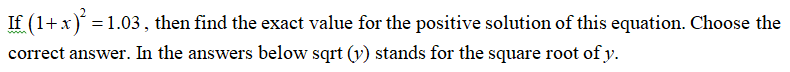# If (1+x)2=1.03(1+x)2=1.03, then find the exact value for the positive solution of this equation. Choose the correct answer. In the answers below sqrt(y) stands for the square root of y.

Question
12 views

If (1+x)2=1.03(1+x)2=1.03, then find the exact value for the positive solution of this equation. Choose the correct answer. In the answers below sqrt(y) stands for the square root of y.

check_circle

Step 1...

### Want to see the full answer?

See Solution

#### Want to see this answer and more?

Solutions are written by subject experts who are available 24/7. Questions are typically answered within 1 hour.*

See Solution
*Response times may vary by subject and question.
Tagged in